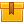# Three-Phase AC Circuits (A8203)

## Prerequisites

This lesson is designed for participants familiar with AC circuits and AC voltage. A basic understanding of how to interpret changes in AC voltage over time is also required.

## Description

This lesson shows how three-phase AC voltage is generated and describes different characteristics of three-phase voltage. The lesson introduces two types of winding electrical connections with graphic demonstrations of their effect on voltage and current. Also presented are transformers and the way in which they affect voltage and current.

## Objectives

Explain how voltage is induced in a three-phase system. Use a sine wave to explain how three-phase voltage changes over time. Describe the effect of three-and four-wire wye connections have on the relationships between phase and line voltage and current in a three-phase system. Describe the effect of a delta connection on the relationship between phase and line voltage and current in a three-phase system. Identify the basic parts of a transformer and describe their functions. Explain what determines how much voltage a transformer produces. Describe how current changes from the primary winding to the secondary winding.

## ContentThis lesson shows how three-phase AC voltage is generated and describes
different characteristics of three-phase voltage. The lesson introduces
two types of winding electrical connections with graphic demonstrations
of their effect on voltage and current.  Also presented are transformers
and the way in which they affect voltage and current.

•Three-phase AC Circuits

0
0 Reviews